# Magnetic Units of Measurement

## DC Electric Circuits

• #### Question 1

In electric circuits, the three basic quantities are voltage (E or V), current (I), and resistance (R), corresponding to the general concepts of cause, effect, and opposition, respectively.

 I = E R

 effect = cause oppositionMagnetic “circuits” also have quantities corresponding to “cause,” “effect,” and “opposition.” Identify these quantities, along with their respective symbols, and write an “Ohm’s Law” equation relating them mathematically. Also, identify the units of measurement associated with each, in three systems of measurement: CGS (“old” metric), SI (“new” metric), and English.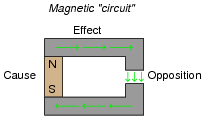• #### Question 2

If we were to graph the “response” of a resistor to various levels of applied voltage, we would obtain a plot that looks like this: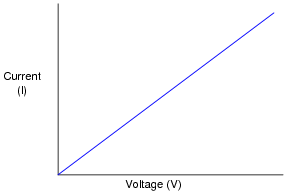If we were to graph the “response” of a ferromagnetic sample to various levels of applied magnetomotive force, we would obtain a plot that looks something like this: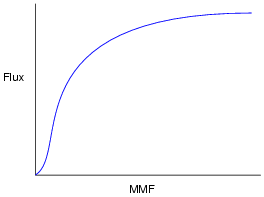What does this graph indicate to you, as compared to the graph for a resistor’s characteristics? What is the significance of this with regard to magnetic “circuit” analysis?

• #### Question 3

Suppose that a length of electrical wire is wrapped around a section of an iron torus, and electric current conducted through the wire: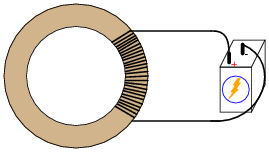What factors influence the amount of MMF, flux, and reluctance in this magnetic “circuit”?

• #### Question 4

Calculate the reluctance (ℜ) for a magnetic circuit where the MMF (F) is 8.9 amp-turns and the flux (Φ) is 0.24 webers.

• #### Question 5

Calculate the amount of magnetic flux (Φ) in a piece of iron with a reluctance (ℜ) of 55 amp-turns per weber and an applied MMF (F) of 2.2 amp-turns.

• #### Question 6

Calculate the amount of magnetomotive force (MMF, or F) required to establish a magnetic flux (Φ) of 30 μWb in a piece of iron having a reluctance (ℜ) of 14 At/Wb.

• #### Question 7

Calculate the amount of MMF (F) generated by a coil of wire having 1300 turns and carrying 3.5 milliamperes of current.

• #### Question 8

Calculate the number of “turns” (wraps) a wire coil would need in order to produce an MMF (F) of 5.7 amp-turns with an electric current of 12 mA.

• #### Question 9

Calculate the amount of electric current that would have to pass through a coil of wire having 850 turns to produce an MMF (F) of 2.1 amp-turns.

• #### Question 10

The formula for calculating the reluctance (ℜ) of an air-core wire coil (“solenoid”) is as follows:

 ℜ = l μ0 A

Where,

l = linear length of coil in meters (m)

A = cross-sectional area of coil “throat” in square meters (m2)

μ0 = permeability of free space = 4 π × 10−7 (T·m/A)

Using this formula and the Rowland’s Law formula, calculate the amount of magnetic flux (Φ) produced in the throat of an air-core solenoid with 250 turns of wire, a length of 0.2 meters, a cross-sectional area of 6.5 × 10−4 square meters, and a coil current of 5 amps: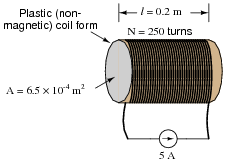• #### Question 11

Electrically conductive materials may be rated according to their relative resistance by a quantity we call specific resistance (ρ). The formula relating resistance to specific resistance looks like this:

 R = ρ l A

Where,

R = Electrical resistance, in ohms

ρ = Specific resistance, in ohm-cmil/ft, or some other combination of units

l = Length of conductor, in feet or cm (depending on units for ρ)

A = Cross-sectional area of conductor, in cmil or cm2 (depending on units for ρ)

Magnetic materials may also be rated according to their relative reluctance by a quantity we call permeability (μ). Write the formula relating reluctance to permeability of a magnetic substance, and note whatever differences and similarities you see between it and the specific resistance formula for electrical circuits.

• #### Question 12

Explain the difference between relative permeability (μr) and absolute permeability (μ). How do the units of measurement differ between these two quantities?

• #### Question 13

Two important variables in magnetic circuit analysis are B and H. Explain what these two variables represent, in terms of the more fundamental magnetic quantities of MMF (F) and flux (Φ), and relate them, if possible, to electrical quantities. Also, determine the units of measurement for these two variables, in the CGS, SI, and English measurement systems.

• #### Question 14

When a steel manufacturer publishes the magnetic characteristics of their latest alloy, they do so in the form of a “B/H” graph, where flux density (B) is plotted as a function of magnetizing force (H):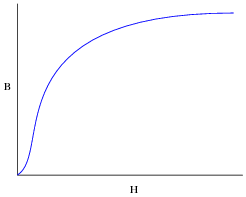Rarely will you see a plot of flux (Φ) shown as as a function of MMF (F), even though such a plot would appear very similar to the “B/H curve” for that same material. Why is this? Why would a “B/H” curve be preferable to an engineer rather than a “Flux/MMF” curve?

Hint: if a copper manufacturer were to publish the electrical characteristics of their latest alloy, they would specify its resistivity in terms of specific resistance (ρ), rather than plain resistance (R), for the exact same reason!

• #### Question 15

Given the following equations, derive a single equation expressing permeability (μ) in terms of flux density (B) and field intensity (H, otherwise known as magnetizing force):

 ℜ = F Φ

 ℜ = l μA

 H = F l

 B = Φ A

• #### Question 16

Using a B-H curve obtained from a reference book, determine the amount of magnetizing force (H) required to establish a magnetic flux density of 0.2 T in a cast iron torus with a cross-sectional area of 7 ×10−4 square meters.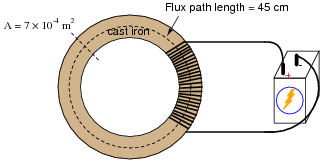Calculate the amount of current necessary in the wire coil to establish this amount of flux, if the coil has 250 turns, and the torus has an average flux path length of 45 cm. Also, calculate the amount of magnetic flux (Φ) inside the torus.